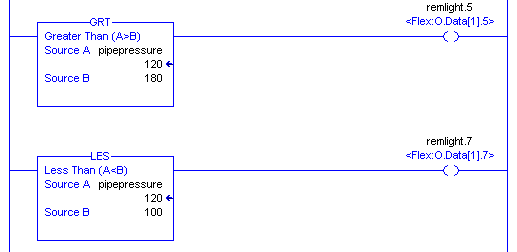# ControlLogix Compare Instructions

## Introduction to ControlLogix Compare Instructions

ControlLogix Compare Instructions are generally used with analog values to determine if a tag is above, below, or within a preset range.   Examples of where a compare instruction might be used is if a tank level exceeds a certain preset.  Also, if a sump level is high, we might use a compare instruction to energize a pump.   Another example would be a speed or amperage of a motor.   We want the operator to see a warning light if a certain preset has been reached.  If you have not done so, please visit the post on Analog Configuration to learn how to set up analog modules so we have values that we can use in this logic.

The compare instructions are found on the “Compare” tab of the instruction toolbar.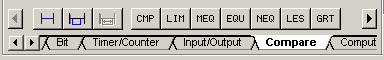## The CMP Instruction

The CMP (Compare) instruction can be used to enter an expression.   If the expression is true in the logic below, the output will energize to indicate that our value is above 180psi.   This output might be a warning to the operator, or even a condition for shutdown.  The CMP instruction can also be used to enter complex math functions that will either return a true or false.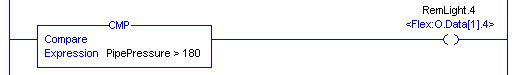## The LIM Instruction

The LIM (Limit) instruction is used to determine if a value is within a certain range.   The Limit is also true AT the limits.   The Low and High Limits can also be tags.   Tags are easier to change than constants (ie from a panelview).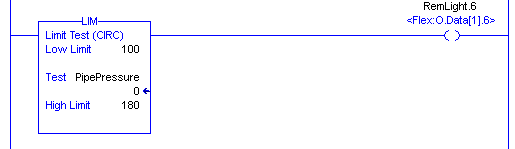In this example, RemLight.6 is OFF because our PipePressure of 0 is not within the limits.   However, if the pipe pressure is between (or at) 100 to 180psi, the light will energize.   We might use this to indicate that the pressure is OK.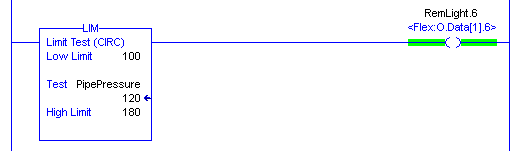Note:  If you reverse the low and the high limits, the instruction works opposite.   The light would be OFF between 100 and 180, but if the PipePressure tags gets outside of the limits, the light would energize.

## The NEQ and EQU instructions

These instructions are opposite of each other.   The EQU instruction will go true when a tag is equal to another value (or tag).  These instructions commonly compare an alarm tag against the value of 0.   There are 32 bits in a DINT tag, and each of the 32 bits might represent an alarm.   If the alarm tag is zero, then we don’t have any alarms.   If the alarm tag becomes a non-zero number, then we might inform the operator that an alarm condition is present.

Here is an example: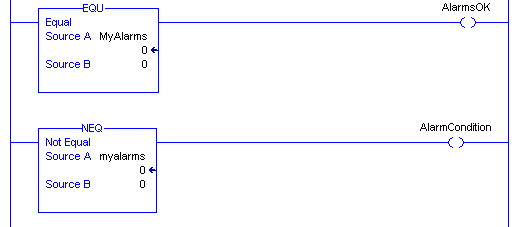## The LES GRT LEQ GEQ Instructions

These instructions are pretty much self-explanatory.   the LES (less than) instruction compares a tag to see if it is less than another value.  GRT (greater than) looks for a tag to be above another value.   LEQ and GEQ (Less than or equal, Greater than or Equal) work the same, except that these instructions also include the exact value that we are comparing a tag against.

Here, remlight.5 will energize if the PipePressure tag exceeds 180.  Remlight.7 will energize if the PipePressure tag falls below 100.